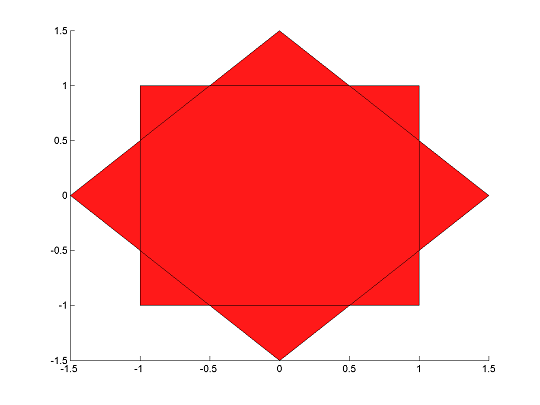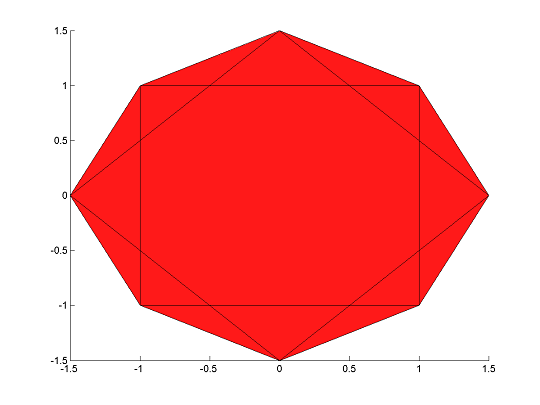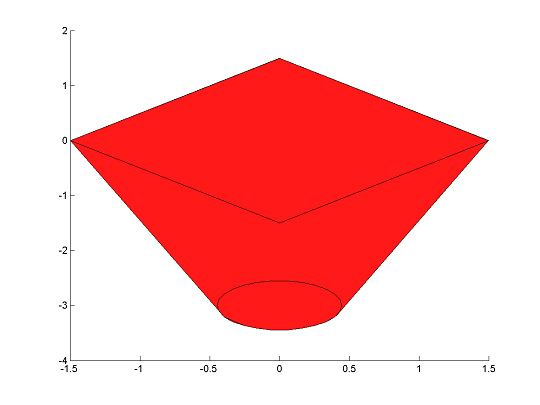hull is used to create the convex hull of a set of constraints.

## Syntax

F = hull(F1,F2,...)


## Examples

Define two polytopes

sdpvar x y
F1 = [-1 <= x <= 1, -1 <= y <= 1];
F2 = [-1.5 <= x-y <= 1.5, -1.5 <= x+y <= 1.5];


Plot the polytopes

plot(F2);hold on
plot(F1);Create a model of the convex hull

H = hull(F1,F2)
+++++++++++++++++++++++++++++++++++++++++++++++++++
|   ID|      Constraint|                      Type|
+++++++++++++++++++++++++++++++++++++++++++++++++++
|   #1|   Numeric value|          Element-wise 2x1|
|   #2|   Numeric value|          Element-wise 2x1|
|   #3|   Numeric value|          Element-wise 2x1|
|   #4|   Numeric value|          Element-wise 2x1|
|   #5|   Numeric value|   Equality constraint 2x1|
|   #6|   Numeric value|   Equality constraint 1x1|
|   #7|   Numeric value|          Element-wise 2x1|
+++++++++++++++++++++++++++++++++++++++++++++++++++


Important to realize is that the representation will introduce new variables due to a lifting procedure. Nevertheless, YALMIP will realize that these are auxiliary variables defined internally, so when you plot the hull, the projection to the original user-defined variables will be plotted.

clf;
plot(H);hold on
plot(F2);
plot(F1);The command applies to (almost) arbitrary convex constraints.

clf;
sdpvar x y
F1 = [1 x y+3;[x;y+3] 1/5*eye(2)] >= 0];
F2 = [-1.5 <= x-y <= 1.5,-1.5 <= x+y <= 1.5];
H = hull(F1,F2);
plot(H,[x y]);hold on
plot(F2);
plot(F1);At the moment, the command does not support constraints that involve nonlinear expression (beyond quadratic or graph-represented).